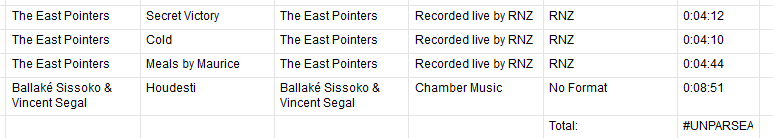Adding time durations as actual numbers

08/15/18 Edited 12/09/19

Hello,

We're trying to use Smartsheet to report on music details.  It would be very useful to take the durations of music tracks (formatted either as mm:ss or h:mm:ss) and add them together together for a total duration.   How would we attempt this?• If you locate successive tracks as children tasks connected to each other by a FS link (Finish-Start, i.e. the standard link between tasks)

Then position a parent task on top of these → the parent will sum up the all sequence automatically.

Alternatively, you can use "+" or "=SUM(...)"

(Sorry I might have missed something because my answer looks too obvious. Do not hesitate to address more constraints if any)

Respectfully yours,

• Unfortunately Smartsheet is not capable of calculating time as far as hours/minutes/seconds go. There are a few workarounds out there in some other threads, but they are all rather tedious and complex.

thinkspi.com

• Since your data is formatted like this

[HHH]H:MM:SS

(assuming hours could be more than 9)

this formula will give you the seconds of a single cell:

=VALUE(LEFT([email protected], FIND(":", [email protected]) - 1)) * 3600 + VALUE(MID([email protected], FIND(":", [email protected]) + 1, 2)) * 60 + VALUE(RIGHT([email protected], 2))

(My column name is TL)

I would add a column to house this and then sum that column.

Craig

• "I would add a column to house this"

We can call this column "Seconds".

"and then sum that column."

If you put the following formula into a column other than the Seconds column (top row of the TL column in Craig's example is what I suggest), Then it will sum all of the rows in the Seconds column and convert it back to HH:MM:SS.

=INT(SUM(Seconds:Seconds) / 3600) + ":" + IF(INT((SUM(Seconds:Seconds) - (VALUE(INT(SUM(Seconds:Seconds) / 3600)) * 3600)) / 60) < 10, "0" + INT((SUM(Seconds:Seconds) - (VALUE(INT(SUM(Seconds:Seconds) / 3600)) * 3600)) / 60), INT((SUM(Seconds:Seconds) - (VALUE(INT(SUM(Seconds:Seconds) / 3600)) * 3600)) / 60)) + ":" + IF((SUM(Seconds:Seconds) - (VALUE(INT(SUM(Seconds:Seconds) / 3600) * 3600) + VALUE(INT((SUM(Seconds:Seconds) - (VALUE(INT(SUM(Seconds:Seconds) / 3600)) * 3600)) / 60) * 60))) < 10, "0" + (SUM(Seconds:Seconds) - (VALUE(INT(SUM(Seconds:Seconds) / 3600) * 3600) + VALUE(INT((SUM(Seconds:Seconds) - (VALUE(INT(SUM(Seconds:Seconds) / 3600)) * 3600)) / 60) * 60))), (SUM(Seconds:Seconds) - (VALUE(INT(SUM(Seconds:Seconds) / 3600) * 3600) + VALUE(INT((SUM(Seconds:Seconds) - (VALUE(INT(SUM(Seconds:Seconds) / 3600)) * 3600)) / 60) * 60))))

If you're looking to use parent rows to show a total of each child row, you would replace Seconds:Seconds with CHILDREN([email protected]).

thinkspi.com

• Here's a shorter version:

=INT([email protected] / 3600) + ":" + IF(INT(([email protected] - INT([email protected] / 3600) * 3600)) < 600, "0") + INT(([email protected] - INT([email protected] / 3600) * 3600) / 60) + ":" + IF([email protected] - (INT([email protected] / 3600) * 3600) - (INT(([email protected] - INT([email protected] / 3600) * 3600) / 60) * 60) < 10, "0") + ([email protected] - (INT([email protected] / 3600) * 3600) - (INT(([email protected] - INT([email protected] / 3600) * 3600) / 60) * 60))

I assume the [email protected] is the summed value.

Why this is shorter:

IF (a very long formula is less than 10, "0" + a very long formula, a very long formula)

I do this:

IF (a very long formula is less than 10, "0") + a very long formula

Craig

• I assume the [email protected] is the summed value.

In my formula, I used the column you put YOUR formula in and called it "Seconds" since it would convert HH:MM:SS into seconds by row.

With your single conversion column going by row, there wasn't a SUM yet. That's why I used Seconds:Seconds. To sum up all of the cells in your conversion column AND convert it back to HH:MM:SS all at the same time.

I do this:

IF (a very long formula is less than 10, "0") + a very long formula

That's definitely a good idea. It saves on space, and depending on the length of the overall formula, is less likely to exceed the 4,000 characters/cell limit.

I would just copy/paste the "very long formula" to avoid typos. Since it isn't very many keystrokes doing it that way, I didn't even think about doing it your way. Definitely going to try to keep it in mind though.

thinkspi.com

• "Copy / Paste is the work of the Devil" - Craig Williams

Theoretically

A = SUM(a bunch of stuff)

B = use A a bunch of times

is faster than

B = SUM(a bunch of stuff) a bunch of times.

My personal character limit on formulas is something less than 500. If it is longer than 100, I reevaluate to see what I'm missing.

Craig

•I love copy/paste when I know something is working. I have fat fingers.

And I see what you're doing. You're going ahead and summing up all of the seconds SUM(Seconds:Seconds) and THEN converting it back to HH:MM:SS.

Even breaking it down into 2 separate steps, it's still shorter and only one more extra cell used. This is why I typically defer to you, the Smartsheet Yoda. HAHA!

dchouston: Craig has the best solution (so far). Only look at my posts if you want to learn from my mistakes. Otherwise... You can ignore them.

thinkspi.com

• Your answers aren't mistakes. They get the job done. In this case, one is it working, it is unlikely it will be changed - does anyone need to see DD:HH:MM:SS?

But ...

"Simple is better than complex. Complex is better than complicated." - Tim Peters

I'm going to post the back story on Copy / Paste to my website.

Craig

• Thanks all...Smartsheet really makes you work for it, don't they!

I've got it all working. Now let's see if I can explain it to radio hosts...

Cheers,
David
• Good luck! Haha.

thinkspi.com